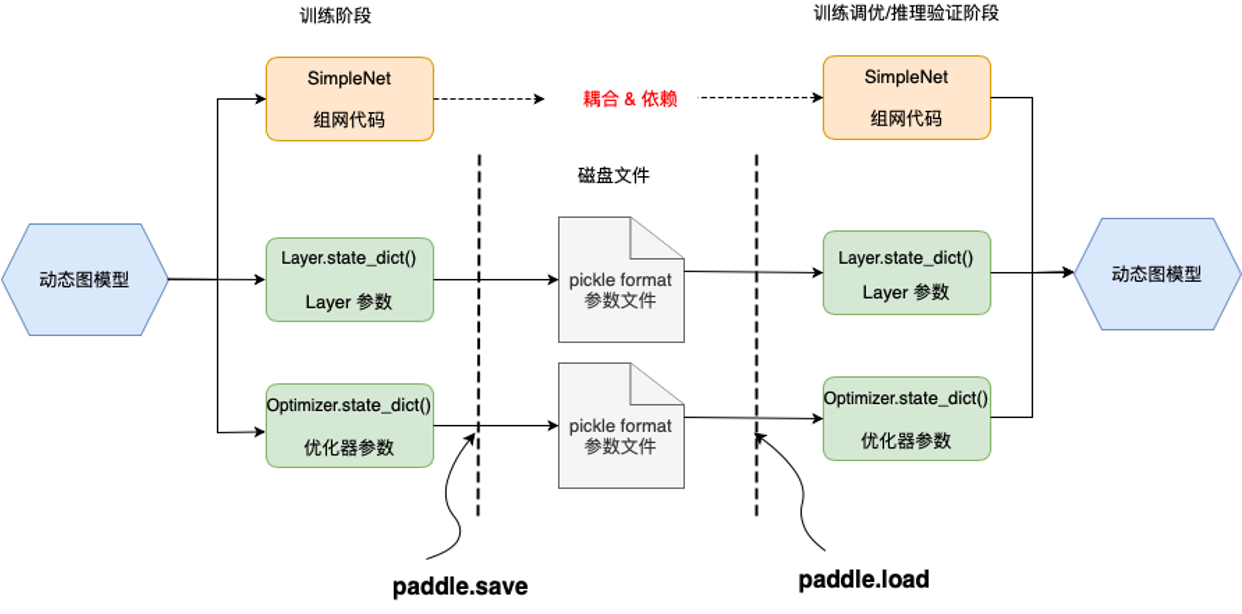# 模型保存与加载¶

## 一、概述¶

• 训练调优场景：

• 模型训练过程中定期保存模型，以便后续对不同时期的模型恢复训练或进行研究；

• 模型训练完毕，需要保存模型方便进行评估测试；

• 载入预训练模型，并对模型进行微调（fine-tune）。

• 推理部署场景：

## 二、用于训练调优场景¶

### 2.1 保存和加载机制介绍¶• paddle.save：使用 `paddle.save`保存模型，实际是通过 Python pickle 模块来实现的，传入要保存的数据对象后，会在指定路径下生成一个 pickle 格式的磁盘文件。

• paddle.load：加载时还需要之前的模型组网代码，并使用`paddle.load`传入保存的文件路径，即可重新将之前保存的数据从磁盘文件中载入。

• 网络层参数`Layer.state_dict()`

• 优化器参数`Optimizer.state_dict()`

• Tensor 数据 ：（如创建的 Tensor 数据、网络层的 weight 数据等）

• 含 Tensor 的 list/dict 嵌套结构对象 （如保存 state_dict() 的嵌套结构对象：`obj = {'model': layer.state_dict(), 'opt': adam.state_dict(), 'epoch': 100}`

### 2.2 使用基础 API¶

```import numpy as np

BATCH_SIZE = 16
BATCH_NUM = 4
EPOCH_NUM = 4

IMAGE_SIZE = 784
CLASS_NUM = 10

final_checkpoint = dict()

# 定义一个随机数据集
def __init__(self, num_samples):
self.num_samples = num_samples

def __getitem__(self, idx):
image = np.random.random([IMAGE_SIZE]).astype('float32')
label = np.random.randint(0, CLASS_NUM - 1, (1, )).astype('int64')
return image, label

def __len__(self):
return self.num_samples

class LinearNet(nn.Layer):
def __init__(self):
super(LinearNet, self).__init__()
self._linear = nn.Linear(IMAGE_SIZE, CLASS_NUM)

def forward(self, x):
return self._linear(x)

for epoch_id in range(EPOCH_NUM):
for batch_id, (image, label) in enumerate(loader()):
out = layer(image)
loss = loss_fn(out, label)
loss.backward()
opt.step()
print("Epoch {} batch {}: loss = {}".format(
epoch_id, batch_id, np.mean(loss.numpy())))
# 最后一个epoch保存检查点checkpoint
if epoch_id == EPOCH_NUM - 1:
final_checkpoint["epoch"] = epoch_id

final_checkpoint["loss"] = loss

# 创建网络、loss和优化器
layer = LinearNet()
loss_fn = nn.CrossEntropyLoss()

dataset = RandomDataset(BATCH_NUM * BATCH_SIZE)
batch_size=BATCH_SIZE,
shuffle=True,
drop_last=True,
num_workers=2)

# 开始训练
```

#### 2.2.1 保存动态图模型¶

```# 保存Layer参数
# 保存优化器参数
# 保存检查点checkpoint信息
```

1. 对于 `Layer.state_dict()` （模型参数），推荐使用后缀 `.pdparams`

2. 对于 `Optimizer.state_dict()` （优化器参数），推荐使用后缀 `.pdopt`

#### 2.2.2 加载动态图模型¶

```# 载入模型参数、优化器参数和最后一个epoch保存的检查点

layer.set_state_dict(layer_state_dict)

# 打印出来之前保存的 checkpoint 信息
print("Loaded Final Checkpoint. Epoch : {}, Loss : {}".format(final_checkpoint_dict["epoch"], final_checkpoint_dict["loss"].numpy()))
```

### 2.3 使用高层 API¶

#### 2.3.1 保存动态图模型¶

```import paddle

parameters=model.parameters())

transform = T.Compose([
T.Transpose(),
T.Normalize([127.5], [127.5])
])

#方式一：设置训练过程中保存模型
model.fit(data, epochs=1, batch_size=32, save_freq=1)

#方式二：设置训练后保存模型
model.save('checkpoint/test')  # save for training
```
• 方式一：开启训练时调用的`paddle.Model.fit`函数可自动保存模型，通过它的参数 `save_freq`可以设置保存动态图模型的频率，即多少个 epoch 保存一次模型，默认值是 1。

• 方式二：调用 `paddle.Model.save`API。只需要传入保存的模型文件的前缀，格式如 `dirname/file_prefix` 或者 `file_prefix` ，即可保存训练后的模型参数和优化器参数，保存后的文件后缀名固定为 `.pdparams``.pdopt`

#### 2.3.2 加载动态图模型¶

```import paddle

parameters=model.parameters())

transform = T.Compose([
T.Transpose(),
T.Normalize([127.5], [127.5])
])
# 加载模型参数和优化器参数
model.fit(data, epochs=1, batch_size=32, save_freq=1)

model.save('checkpoint/test_1')  # save for training
```

## 三、用于推理部署场景¶

### 3.2 使用高层 API¶

`paddle.Model.save`的第一个参数需要设置为待保存的模型和参数等文件的前缀名，第二个参数 `training` 表示是否保存动态图模型以继续训练，默认是 True，这里需要设为 False，即保存推理部署所需的参数与文件。接前文高层 API 训练的示例代码，保存推理模型代码示例如下：

```model.save('inference_model', False)  # save for inference
```

```inference_model.pdiparams        // 存放模型中所有的权重数据
inference_model.pdmodel         // 存放模型的网络结构
inference_model.pdiparams.info   // 存放和参数状态有关的额外信息
```

## 四、其他场景¶

### 4.2 静态图模型的保存与加载¶

• 若仅需要保存/载入模型的参数用于训练调优场景，可以使用 `paddle.save`/`paddle.load` 结合静态图模型 Program 的 state_dict 达成目的。也支持保存整个模型，可以使用 `paddle.save` 将 Program 和state_dict 都保存下来。高层 API 兼容了动态图和静态图，因此`Paddle.Model.save``Paddle.Model.load`也兼容了动、静态图的保存和加载。

• 若需保存推理模型用于模型部署场景，则可以通过 `paddle.static.save_inference_model``paddle.static.load_inference_model`实现。

#### 4.2.1 训练调优场景¶

```import paddle
# 开启静态图模式

# 创建输入数据和网络
x = paddle.static.data(name="x", shape=[None, 224], dtype='float32')

# 设置执行器开始训练
```

```# 保存模型参数
```

```# 保存模型结构（program）
```

```# 载入模型结构（program）
```

```# 载入模型参数
prog.set_state_dict(state_dict)
```

#### 4.2.2 推理部署场景¶

```import paddle
import numpy as np
# 开启静态图模式

# 创建输入数据和网络
# 设置执行器开始训练
exe.run(startup_prog)
```

```# 保存静态图推理模型
path_prefix = "./infer_model"
```

```# 载入静态图推理模型
[inference_program, feed_target_names, fetch_targets] = (
• 如果用于训练调优场景，动态图和静态图均使用 `paddle.save``paddle.load`保存和加载模型参数，或者在高层 API 训练场景下使用 `paddle.Model.save``paddle.Model.load`
• 如果用于推理部署场景，动态图模型需先转为静态图模型再保存，使用 `paddle.jit.save``paddle.jit.load`保存和加载模型结构和参数；静态图模型直接使用 `paddle.static.save_inference_model``paddle.static.load_inference_model`保存和加载模型结构和参数。